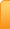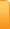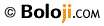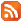Hand Multiplication - 2 by N. S. MurtyChannelsAnalysis
Cartoons
Education
Environment
Opinion
Photo Essays

Random Thoughts

Architecture
Astrology
Ayurveda
Buddhism
Cinema
Culture
Festivals
Hinduism
History
People
Places
Sikhism
Spirituality

Health
Parenting
Perspective
Recipes
Society
Teens
Women

## Creative Writings

Book Reviews
Computing
Humor
Individuality
Literary Shelf
Memoirs
Quotes
Stories
Travelogues
Workshop

Hand Multiplication - 2
by N. S. Murty

Continued from Previous Page

One of my friends in his feedback told me that he is not able to get the value for 15 multiplied by 15 by the method I explained in my last article. He made a small mistake and that is why he did not get the answer.

Perhaps, some of the other friends might have also experienced a similar situation though they did not give any feedback.

Recap:

Let me explain the procedure once again. This procedure explained by me is applicable when we are multiplying two numbers.

We assign values to the right and left hands. Values start from little finger upwards to Thumb and the Little finger (LL or RL) will have either 1 or 6 in its units place, the Ring finger (LR or RR) will have 2 or 7 in units place, Middle finger (LM or RM) will have 3 or 8 in units place, Index finger (LI or RI) will have 4 or 9 in units place and the Thumb (LT or RT) will have 0 or 5 in units place.

Procedure:

Step 1:

We just touch (or join) the corresponding fingers as the multiplicands. That is, for 17 x 18. We join LR and RM.

Step 2:

Assigning Values:

Component 1: Base values: All fingers touching and lying below will have specific values depending upon the multiplicands. For convenience I am providing in the table below for easy practice. But as you do the multiplication repeatedly, you will know it automatically. The very purpose of this article is to know the underlying principle than getting values by heart.

Component 2: Values of Free Fingers: In each multiplication there will be Free fingers, that means, fingers lying above the ‘fingers touched or joined) or none (when thumb is one of the fingers joined). These Free fingers also will have their corresponding values depending upon the multiplicands.

The Final value is always the sum of the two components.

Very Important Note:

Once we join the fingers for multiplication, we ignore the values assigned to the respective fingers. We now embark upon eliciting the value of product and treat them as ‘Fingers joined’ and ‘Free fingers’ only.

Coming to the given example 17 x 18, once we join LR and RM, we try to evaluate the product treating LL, LR, RL, RR and RM as 5 fingers and the fingers on the left and right hand as 3 free fingers and 2 free fingers respectively.

How to assign the Base value?

The Base value will always be the sum of the values assigned to little fingers minus 2. (In fact it is the sum of the numbers preceding the value of little fingers).

Let me explain by example:

When we multiply 17 and 18, we assign 16 to the little fingers on each hand. Therefore, the base value for this multiplication is, all fingers touching or lying below them will be 30.

Similarly, if you multiply 12 by 18, the Base value will be 10 + 15= 25

If you multiply 6 by 19, the base value will be 5 + 15= 20.

And so on.

How to Assign Values to Free fingers?

Remember, we are multiplying numbers with our hands each of which has only 5 fingers. So we are doing multiplications in cycles of 5.

Let me call the cycle 6 to 10 (that is 6x6 to 10 x 10) as cycle 1.

In Cycle 1, all ‘Free fingers’ represent only Units.

In Cycle 2, when the numbers start from 11 to 15, we add 5 to the number of free fingers on the opposite hand.

In cycle 3, when the numbers start from 16 to 20, we add 10 to the number of free fingers on the opposite hand.

As a corollary, when two hands are in different cycles, the free fingers will have the values depending upon the cycle in which the numbers of the opposite hand represent.

Coming to the multiplication we started, 17 x 18, the base value is 30 and the value of free fingers on the left is 13 (3+10) and on the right is 12 (2+10).

So the Component 1 of the product is 30 x 5= 150.

Component 2 of the product is 12x 13= 156

And the final value of the product is 150 + 156 = 306

Table of values for Base and free fingers

 S. No Cycle of the Left Hand Cycle of the Right Hand Base value Value of Free fingers on Left Hand Value of Free fingers on Right Hand 1 1(6 to 10) 1(6 to 10) 5+5= 10 Units Units 2 1(6 to 10) 2(11 to 15) 5+10=15 +5 3 2(11 to 15) 2(11 to 15) 10+ 10=20 +5 +5 4 2(11 to 15) 3(16 to 20) 10+15= 25 +10 +5 5 3(16 to 20) 3(16 to 20) 15+15= 30 +10 +10

Note: When the hands are in cycles 2 and above and LT or RT are being used, you may think there are no free fingers hence the value is zero. No. You still have to add the corresponding value depending upon the cycle in which the other hand is and then proceed to evaluate component 2.

Here is the corresponding table when each hand is in different cycles

 S. No Cycle of the Left Hand Cycle of the Right Hand Base value Value of Free fingers on Left Hand Value of Free fingers on Right Hand 6 1(6 to 10) 3(16 to 20) 5+15= 20 +10 Units 7 1(6 to 10) 4(21to 25) 5+20=25 +15 Units 8 2(11 to 15) 4(21 to 25) 10+20= 30 +15 +5

Example for S. Nos. 6

8 x 19

Base value: 5 + 15 = 20

Component 1: 7 x 20 = 140

Component 2: 12 x 1= 12

Product: 140 + 12= 152

Example for S. No. 7

9 x 23

Base value: 5+ 20 = 25

Component 1: 7 x 25= 175

Component 2: 16x 2= 32

Product: 175 + 32= 207

Example for S. No 8

13 x 23

Base Value: 10+ 20 = 30

Component 1: 6 x 30 = 180

Component 2: 17 x 7 = 119

Product: 180 + 119= 299

Coming back to where I started, my friend did not get the correct value for 15 x 15 and 20 x 20 because… you guessed it right; he forgot to add the corresponding values and treated Component 2 as zero in each case.

In fact Component 2 in 15 x 15 is 5 x 5= 25 (Component 1 being 10 x 20= 200)

And Component 2 in 20 x 20 is 10 x 10= 100 (Component 1 being 10x 30= 300)

Try to practice regularly with little patience. You are sure to master the technique. In competitive examinations, where calculators are not allowed, this will come in handy. And as you grow up, and need to calculate your investments, insurances, interest components etc., it will be a handy tool.

17-Nov-2018
More by :  N. S. Murty

Top | Education

Views: 1036      Comments: 0

Name *
Email ID
(will not be published)
Comment *
Characters
Verification Code*
Please fill the above code for verification.RSS Feed Home | Privacy Policy | Disclaimer | Site Map